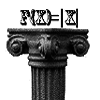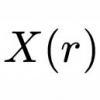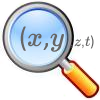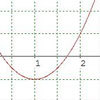# Resources tagged with: Calculus generally

Filter by: Content type:
Age range:
Challenge level:

### There are 27 results

Broad Topics > Calculus > Calculus generally### Maths Shop Window

##### Age 16 to 18 Challenge Level:

Make a functional window display which will both satisfy the manager and make sense to the shoppers### Scientific Curves

##### Age 16 to 18 Challenge Level:

Can you sketch these difficult curves, which have uses in mathematical modelling?### Power Up

##### Age 16 to 18 Challenge Level:

Show without recourse to any calculating aid that 7^{1/2} + 7^{1/3} + 7^{1/4} < 7 and 4^{1/2} + 4^{1/3} + 4^{1/4} > 4 . Sketch the graph of f(x) = x^{1/2} + x^{1/3} + x^{1/4} -x### Patterns of Inflection

##### Age 16 to 18 Challenge Level:

Find the relationship between the locations of points of inflection, maxima and minima of functions.### Implicitly

##### Age 16 to 18 Challenge Level:

Can you find the maximum value of the curve defined by this expression?### Fractional Calculus II

##### Age 16 to 18

Here explore some ideas of how the definitions and methods of calculus change if you integrate or differentiate n times when n is not a whole number.### Calculus Countdown

##### Age 16 to 18 Challenge Level:

Can you hit the target functions using a set of input functions and a little calculus and algebra?### Operating Machines

##### Age 16 to 18 Challenge Level:

What functions can you make using the function machines RECIPROCAL and PRODUCT and the operator machines DIFF and INT?### Curvy Catalogue

##### Age 16 to 18 Challenge Level:

Make a catalogue of curves with various properties.### Fractional Calculus I

##### Age 16 to 18

You can differentiate and integrate n times but what if n is not a whole number? This generalisation of calculus was introduced and discussed on askNRICH by some school students.### Calculus Analogies

##### Age 16 to 18 Challenge Level:

Consider these analogies for helping to understand key concepts in calculus.### Population Dynamics - Part 2

##### Age 16 to 18 Challenge Level:

Second in our series of problems on population dynamics for advanced students.### Population Dynamics Collection

##### Age 16 to 18 Challenge Level:

This is our collection of tasks on the mathematical theme of 'Population Dynamics' for advanced students and those interested in mathematical modelling.### Population Ecology Using Probability

##### Age 16 to 18 Challenge Level:

An advanced mathematical exploration supporting our series of articles on population dynamics for advanced students.### Population Dynamics - Part 6

##### Age 16 to 18 Challenge Level:

Sixth in our series of problems on population dynamics for advanced students.### Population Dynamics - Part 5

##### Age 16 to 18 Challenge Level:

Fifth in our series of problems on population dynamics for advanced students.### Population Dynamics - Part 4

##### Age 16 to 18 Challenge Level:

Fourth in our series of problems on population dynamics for advanced students.### Population Dynamics - Part 3

##### Age 16 to 18 Challenge Level:

Third in our series of problems on population dynamics for advanced students.### A Different Differential Equation

##### Age 16 to 18 Challenge Level:

Explore the properties of this different sort of differential equation.### Population Dynamics - Part 1

##### Age 16 to 18 Challenge Level:

First in our series of problems on population dynamics for advanced students.### Branching Processes and Extinction

##### Age 16 to 18 Challenge Level:

An advanced mathematical exploration supporting our series of articles on population dynamics for advanced students.### Integral Arranging

##### Age 16 to 18 Challenge Level:

How would you sort out these integrals?### Fractional Calculus III

##### Age 16 to 18

Fractional calculus is a generalisation of ordinary calculus where you can differentiate n times when n is not a whole number.### Interactive Workout - Mathmo

##### Age 16 to 18 Short Challenge Level:

Mathmo is a revision tool for post-16 mathematics. It's great installed as a smartphone app, but it works well in pads and desktops and notebooks too. Give yourself a mathematical workout!### Turning to Calculus

##### Age 16 to 18 Challenge Level:

Get started with calculus by exploring the connections between the sign of a curve and the sign of its gradient.